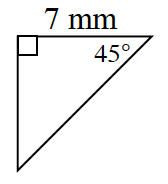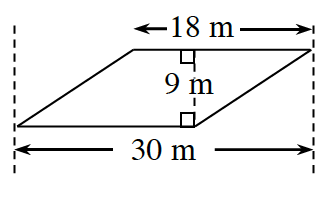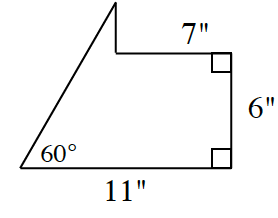### Home > INT2 > Chapter 11 > Lesson 11.1.2 > Problem11-25

11-25.

Calculate the perimeter of each shape below. Assume the diagram in part (b) is a parallelogram.

1.This is a $45°$ - $45°$ - $90°$ triangle. The other side is therefore $7$ mm and the hypotenuse is $7\sqrt{ 2}$ mm.

1.The unknown sides both have the same length and are the hypotenuses of special right triangles which have the side ratio $3$-$4$-$5$.

1.$P ≈ 32.9\; \text{in.}$